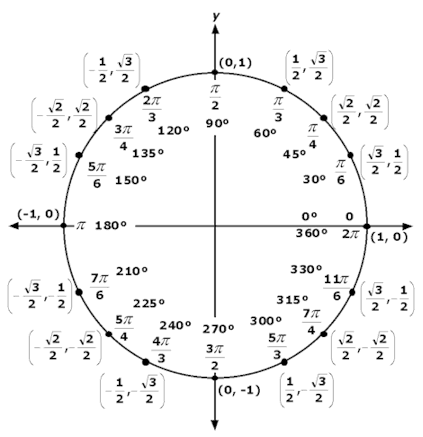×#### Thank you for registering.

One of our academic counsellors will contact you within 1 working day.

Click to Chat

1800-1023-196

+91-120-4616500

CART 0

• 0

MY CART (5)

Use Coupon: CART20 and get 20% off on all online Study Material

ITEM
DETAILS
MRP
DISCOUNT
FINAL PRICE
Total Price: Rs.

There are no items in this cart.
Continue Shopping```Trigonometric RatiosTrigonometric ratios provide relationship between the sides and angles of a right angled triangle.

The plane is basically divided into four quadrants by the rectangular co-ordinate axis. An angle is said to be positive if it is measured in anti-clockwise direction from the positive x- axis and similarly, it is considered to be negative if it is measured in the clockwise direction.In Fig. 1, note that

∠xoy = π/2, ∠xox’ = π, ∠xoy’ = 3π/2.

Here PiQi denote the y coordinate and hence are positive whenever above the x axis, while it is negative when below the x- axis. Though OPi is always taken as positive, OQi is positive only in the positive direction of x- axis and negative when along the negative direction.

The trigonometric formulas are easy to memorize and fetch some direct questions. Various trigonometric ratios have different signs depending on the quadrant they lie in.

Some Important points

Two angles whose sum is 90o are called complementary angles while those   whose sum equals 180o are called supplementary angles.

Since the trigonometric ratios are ratio between two sides of a right angled triangle with respect to an angle, so they are real numbers.

The domain of sine and cos is R, i.e. the real numbers while their value varies from -1 to 1.

The domain of tan x is R – {(2n + 1) π/2 , n ∈ I} while its range is R.

The domain of cosec x is R – {nπ, n ∈ I} while its range is R – {x: -1 < x < 1}.

The domain of cot x is R – {nπ, n ∈ I} while its range is R.

The domain of sec x is R – {(2n + 1) π/2 , n ∈ I} while its range is R except {x: -1 < x < 1}.

sin (nπ + (–1)n) θ = sin θ, n ∈ I

cos (2nπ + π) = cos π, n ∈ I.

tan (nπ + π) = tan π, n ∈ I

Watch this Video for more reference

Given below is a trigonometric ratios chart which depicts the values of sin and cosine functions at different angles:Illustration:

Given A = sin2θ + cos4θ, then for all real values of

(a) 1 ≤ A ≤ 2

(b) 3/4 ≤ A ≤ 1

(c) 13/16 ≤ A ≤ 1

(d) 3/4 ≤ A ≤ 13/16

Solution:  Given A = sin2θ + cos4θ

= sin2θ + (1 - sin2θ)2

Hence, A = sin4θ - sin2θ +1.

This gives A = (sin2θ – 1/2)2 + 3/4

Since 0 ≤ sin2θ ≤ 1

Hence, 0 ≤ (sin2θ – 1/2)2 ≤ 1/4

Therefore, 3/4 ≤ A ≤ 1.

Illustration:

The expression

3[sin4 (3π/2 – α) + sin4 (3π + α)] – 2[sin6 (π/2 + α) + sin6 (5π – α)] is equal to

(a) 0

(b) 1

(c) 3

(d) sin 4α + cos 6α

Solution:

The given expression is

3[sin4 (3π/2 – α) + sin4 (3π + α)] – 2[sin6 (π/2 + α) + sin6 (5π – α)]

= 3(cos4 α + sin4 α) -2 (cos6 α + sin6 α)

= 3(1-2 cos2 α sin2 α) -2(1-3 cos2 α sin2 α)

= 3-6 cos2 α sin2 α – 2 + 6 cos2 α sin2 α

= 1.

Related Resources

Look into the Revision Notes on Trigonometry for a quick revision.

Various Recommended Books of Mathematics are just a click away.

To read more, Buy study materials of Inverse Trigonometric Functions comprising study notes, revision notes, video lectures, previous year solved questions etc. Also browse for more study materials on Mathematics here.
```### Course Features

• 731 Video Lectures
• Revision Notes
• Previous Year Papers
• Mind Map
• Study Planner
• NCERT Solutions
• Discussion Forum
• Test paper with Video Solution